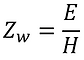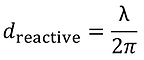top of page

## 6  Electromagnetic Fields

EMI often happens due to electromagnetic radiation (see coupling paths). In order to reduce the radiated coupling, it is important to understand electromagnetic radiation and the implications of the near-field and the far-field.

The Electromagnetic Field.

The electromagnetic field can be seen as the combination of an electric field E [V/m] and a magnetic field H [A/m] traveling in the same direction. The figure below shows an electromagnetic field in form of a plane wave, where the electric field and the magnetic field are perpendicular to each other.The Poynting vector S [W/m^2] represents the direction of propagation of an electromagnetic wave and the power density vector of a radiated electromagnetic field in [W/m^2]. S is defined as the cross product of the two vector fields E and H  [6.5]:where S [W/m^2] is the directional energy flux vector (the energy transfer per unit area per unit time) of an electromagnetic field, E [V/m] is the electric field vector, and H* [A/m] is the complex conjugate of the magnetic field vector H [A/m].

Instead of working with complex field vectors, it is often much more practical to work with the average power density Savg [W/m^2]. For a uniform plane wave (electromagnetic field in the far-field, where the wave impedance Zw [Ω] is equal to the intrinsic impedance η [Ω]) in a lossless medium (εr = 1:0 and µr = 1:0), the calculation of the average power density Savg [W/m^2] can be simplified to:where E [V/m] is the amplitude/magnitude of the electric field vector/phasor, H [A/m] is the amplitude/magnitude of the magnetic field vector/phasor and η [Ω] is the intrinsic impedance of the medium where the uniform plane wave is traveling through. In the equation above, the factor 1/2 can be omitted for RMS values of the E-field and the H-field. For free-space, η [Ω] can be set to η0 = 377Ω.

Electromagnetic Field Characteristics.

An electromagnetic field is characterized by three things [6.4]:

1. Source. The antenna (e.g. dipole/horn/loop antenna, cable, PCB trace).

2. Media. The media surrounding the source (e.g. air, plastics, metal).

3. Distance. The distance between the source and the observation point. Close (compared to the wavelength λ) to the source, the field properties are determined primarily by the source characteristics (wave impedance Zw). Far from the source, the field depends mainly on the medium through which the field is propagating.
Therefore, the space around a source of radiation can be split into two regions: The near-field and the far-field.

For EMC emission measurements, it is important to know if a certain measurement takes place in the near-field or the far-field. Different probes and antennas have to be used accordingly.

• Near-field. Special near-field probes are used to measure the H- or E-field.

• Far-field. Log-periodic, biconical or horn antennas are used to measure the EM-field.

Wave Impedance.

Before we dive into the near- and far-field topic, we have to talk about the wave impedance. The wave impedance is the characteristic of a medium (air, transmission line dielectric medium, etc.) in which a wave propagates.

Why is it important to know the characteristic impedance? Because the ratio of reflected and transmitted amplitude of the wave at an interface, from one medium to another, is determined by the characteristic impedance of the two media.

For ANY electromagnetic wave, the wave impedance ZW in [Ω] is defined as [6.1]:E is the electric field in [V/m] and H is the magnetic field in [A/m].

The characteristic impedance Z0 [Ω] of a medium (NOT a transmission line, here we talk about electromagnetic waves traveling through a medium like air, insulator, metal shield, etc.) is defined as [6.1]:ω=angular frequency [rad/sec] of the signal, μ=permeability of the medium [H/m], ε=dielectric constant of the medium [F/m], σ=conductivity of the medium [S/m].

For insulators and air (σ << jωε) the characteristic impedance is independent of the signal frequency [6.1]:μ=permeability of the medium [H/m], ε=dielectric constant of the medium [F/m], μ0=permeability of free-space 4πE-7 [H/m], μr=relative permeability of the media , ε0=dielectric constant of free-space 8,85E-12 [F/m], εr=relative dielectric constant of the media.

For free-space (and approximately for air) are: μr=1, εr=1. Therefore, the characteristic impedance of free-space (air) is defined as [6.1]:Wave Impedance vs. Distance.

The graph below shows the wave impedance ZW [Ω] in dependency of the distance d [m] to the source (normalized with λ/(2*π)) for an ideal Hertzian dipole (an infinitesimal element of length dl). The graph is a simplification and it should just illustrate how the magnetic field H [A/m] and electric field E [V/m] differ in their wave impedance ZW [Ω] in the near field and that the electromagnetic field (EM-field) has a constant wave impedance ZW [6.1].Near-Field to Far-Field Boundary.

The electromagnetic field around an antenna can be divided in three regions [6.2]:

1. Reactive near-field

3. ​Far-field

The regions depend on the maximum linear dimension of the antenna D [m] and the wavelength of the signal λ [m]:

• D<λ/2. Electrically small antennas. The reactive near-field is significant. For electrically small antennas, the radiating near-field and the far-field are minimal if they exist at all.

• D>λ/2. Electrically large antennas. All three regions are significant: the reactive near-field, the radiating near-field and the far-field.Near-Field.

In the near-field, the characteristic impedance depends primarily on the source and the electric and magnetic fields have to be considered separately (because the ratio of E/H is not constant).

• The electric field E is predominant in these cases [6.3]:

• The source voltage is high compared to the source current (E/H > 377Ω).​

• The source impedance is high (e.g. dipole or straight wire antennas).

• As a consequence of a predominant E-field:​​​​

• The wave impedance near the antenna is high.

• E-field attenuates with a rate of 1/d^3 in the near field (d = distance to source).

• H-field attenuates with a rate of 1/d^2 in the near field (d = distance to source).

• The magnetic field H is predominant in these cases [6.3]:

• The source voltage is low compared to the source current (E/H < 377Ω).​

• The source impedance is low (e.g. loop antennas).

• ​As a consequence of a predominant H-field:

• The wave impedance near the antenna is low.

• E-field attenuates with a rate of 1/d^2 in the near field (d = distance to source).

• H-field attenuates with a rate of 1/d^3 in the near field (d = distance to source).

As mentioned above, the near-field can be divided into the following two regions:

• Reactive Near-Field. In the reactive near-field, energy is stored in the electric and magnetic fields very close to the source, but not radiated from them. Instead, energy is exchanged between the signal source and the fields. In the case of D<λ/2, the reactive near-field extends until the distance d [m] from the antenna by [6.2]:where λ is the wavelength [m] of the signal. In case of D>λ/2, the reactive near-field extends until the distance d [m] from the antenna by [6.2]:where λ is the wavelength [m] of the signal and D the maximum linear dimension of the antenna [m].

• Radiating Near-Field (Fresnel Zone). In the radiative or radiating near-field, the angular field distribution depends on distance d from the antenna, unlike in the far-field where it does not depend on the distance. Energy is radiated as well as exchanged between the source and a reactive near-field. In case of D>λ/2, the radiating near-field extends until the distance d [m] from the antenna by [6.2]:where λ is the wavelength [m] of the signal and D the maximum linear dimension of the antenna [m]. Beyond the radiating near-field, the far-field begins.

Far-Field (Fraunhofer Region).

In the far-field, the E- and H-fields move perpendicular (orthogonal) and in phase to each other and form a plane wave.

• E- and H-fields drop with a rate of 1/d in the far field (d = distance to source).

• The wave impedance in free-space (air) is 377Ω.

There is usually only a far-field region in the case of D>λ/2, where λ is the wavelength [m] of the signal and D is the maximum linear dimension of the antenna [m]. However, if there is a far-field, it starts roughly at the following distance d [m] from the antenna [6.2]:The Electromagntic Field
Electromagnetic Field Characteristic
Wave Impedance
Wave Impedance vs. Distance
Near-Field / Far-Field Boundary
Near-Field
Far-Field
bottom of page